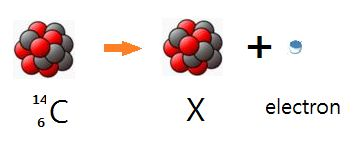# Beta decay of carbon-14

Chemistry Level 1The above is an example of $\beta^{-}$ decay when carbon-$14$ decays into X. Which of the following is the X nucleus above?

×